# Special Relativity: Intuitive Explanation

During the early years of the twentieth century, Albert Einstein revolutionized our understanding of the physical world. In 1905 he proposed his special theory of relativity, which fatally undermined common assumptions about the nature of the space and time. One of the major predictions of the special relativity is the differences in measurement of time and length between observers moving at different relative speeds. Time seems to move slower for objects that are moving faster than us. This is called time dilation. Also, measurement of the same length in the direction of movement is different for observers moving at different speeds. This is called length contraction. The goal of this post is to explain why length contraction and time dilation happens without resorting to complex mathematical derivations, the use of non-intuitive spacetime diagrams, and comparisons with Newtonian physics.

# There is only one speed: speed of light

The main observation that leads to predictions of time dilation and length contraction by Special Relativity is the constancy of light’s speed. This is true, no matter how fast the observer (the agent who is emitting light) moves. Many experiments including famous Michelson-Morely experiment have shown this to be true.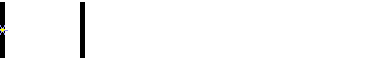Slower displacement than the speed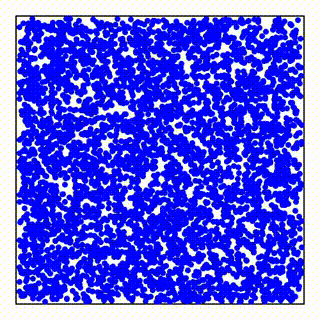Matter composed of confined light speed particles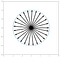In a stationary matter, a particle is equally likely to move in any direction. Each arrow shows a potential velocity a particle can have. Since light speed is the only speed, it is easier to choose the units such that light speed is 1 in all our diagrams.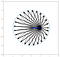In a moving matter, all particle’s speeds should get modified from the point of the view of the observer moving with it.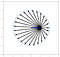If the measurement of time and distance don’t change with changes in speed, we should measure different light speeds in different directions.

# True nature of time and its relation to space

Time is an indicator of change in the universe. If the universe never changes, time never passes. We get old because our body goes through changes and these changes are driven mostly by molecules going through different chemical reactions. Chemical reactions themselves are driven by the interaction of particles of matter with each other. At small enough scale passage of time is the number of interactions particles of matter have with each other. The faster they interact, the faster time goes by.

# Time dilation

As mentioned before, if the measurement of time and length don’t change, a moving matter seems to experience different light speeds in different directions.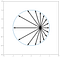What measurement of light speed for a moving observer should be if time dilation or length contraction don’t occur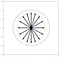Average speed in each direction without time dilation or length contraction. These average speeds give us a skinny ellipse. The blue circle is what the experiment tells us these speeds should be. The constant speed of light in all direction.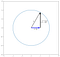Time dilation calculation is simple Pythagoras theorem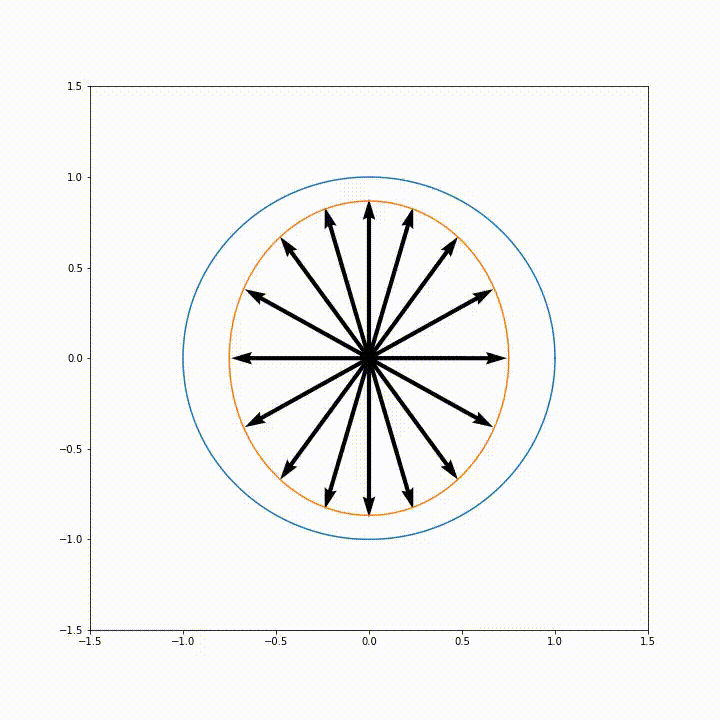time dilation in all directions

# Length contraction

After applying time dilation, speeds parallel to the movement still come out to be less than the speed of light. Length contraction in the direction of movement is needed to match the speed of light. Average speed in a parallel direction to movement without time dilation and length contraction is average of 1+(V/C) and 1-(V/C), which is 1- (V/C)².